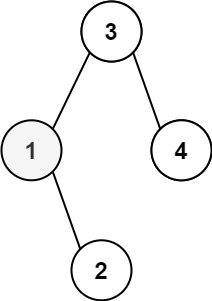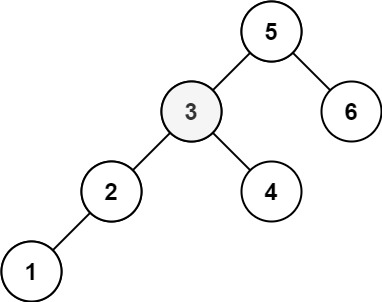# 230. Kth Smallest Element in a BST / Medium

Given the root of a binary search tree, and an integer k, return the kth smallest value (1-indexed) of all the values of the nodes in the tree.

## Example 1:Input: root = [3,1,4,null,2], k = 1
Output: 1

## Example 2:Input: root = [5,3,6,2,4,null,null,1], k = 3
Output: 3

## Comstraints

• The number of nodes in the tree is n.
• 1 <= k <= n <= 10^4
• 0 <= Node.val <= 10^4
• Follow up: If the BST is modified often (i.e., we can do insert and delete operations) and you need to find the kth smallest frequently, how would you optimize?

# Solution 1: Traverse and store

## 效能

### Complexity

• Time Complexity: O(N)
• Space Complexity: O(N)

### LeetCode Result

• Runtime: 20 ms
• Memory Usage: 24.2 MB

# Solution 2: Iterate with Stack

## 效能

### Complexity

• Time Complexity: O(N)
• Space Complexity: O(N)

### LeetCode Result

• Runtime: 21 ms
• Memory Usage: 24.2 MB